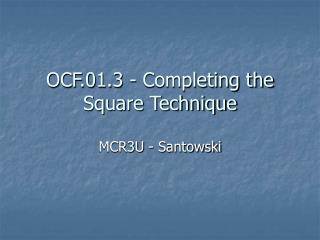# OCF.01.3 - Completing the Square Technique - PowerPoint PPT PresentationDownload PresentationOCF.01.3 - Completing the Square Technique

Download Presentation## OCF.01.3 - Completing the Square Technique

- - - - - - - - - - - - - - - - - - - - - - - - - - - E N D - - - - - - - - - - - - - - - - - - - - - - - - - - -
##### Presentation Transcript

1. OCF.01.3 - Completing the Square Technique MCR3U - Santowski

2. (A) Perfect Square Trinomials • Polynomials like (x + 4)2 are called perfect squares. • When expanded, (x + 4)2 equals x2 + 8x + 16. • When x2 + 8x + 16 is factored, it becomes (x + 4)2 • Notice the relationship between the x term (8) and the constant term (16) ????? • If not, try another perfect square, say (x + 6)2 and x2 + 12x + 36 (12 and 36??) • ex. Find the value of b that makes each expression a perfect square • (a) x2 + 4x + b • (b) x2 + 10x + b • (c) x2 + 14x + b • (d) x2 - 12x + b • (e) x2 – 5x + b

3. (B) The Vertex Form of a Quadratic Equation • The quadratic equation y = a(x – h)2 + k is the vertex form and conveniently gives us the location of the vertex of the parabola, which is at (h,k). • In many cases the equation of a quadratic may be presented in standard form y = ax2 + bx + c • We have to go through the “completing the square” technique in order to convert the equation to the vertex form. • Then, we can identify the vertex and if the vertex represents a maximum (parabola opens down) or a minimum (parabola opens up) by the sign of the a term. If a is positive, the parabola opens up and the vertex represents a min. point and if a is negative, the parabola opens down and the vertex represents a max. point.

4. (C) The Completing the Square Method • Steps Involved in the technique: • Example using y = 2x2 + 12x - 3 • (i) Factor the leading co-efficient of 2 (the value of a) from both the x2 and x terms  y = 2(x2 + 6x) - 3 • (ii) Find the constant term that must be present in order to create a perfect square trinomial  y = 2(x2 + 6x + 9 ) - 3  BUT *****????? • (iii) Make an adjustment in the equation because of the extra value added (+9)  y = 2(x2 + 6x + 9 - 9) - 3 • (iv) Group the perfect square trinomial. Move the subtracted value out of the bracket  y = 2(x2 + 6x + 9) – 2x9 - 3 • (v) Factor the perfect square and collect like terms  y = 2(x + 3)2 - 21

5. (D) Further Examples of the Method • ex 1. y = x2 + 8x + 15 • ex 2. y = -2x2 + 12x - 7 • ex 3. y = -0.3f2 - 2.4f + 7.3 • ex 4. y = 5x – 3x2 • In each example, identify the vertex and the direction of opening of the parabola

6. Internet Links • Completing the Square by James Brennan • Completing the Square: Solving Quadratics from Purple Math • Completing the Square from Bethany Lutheran College

7. (E) Homework • Nelson Text, p306, Q1abcdefg, 2abcdef, 3abc, 5abcd, 7, 9